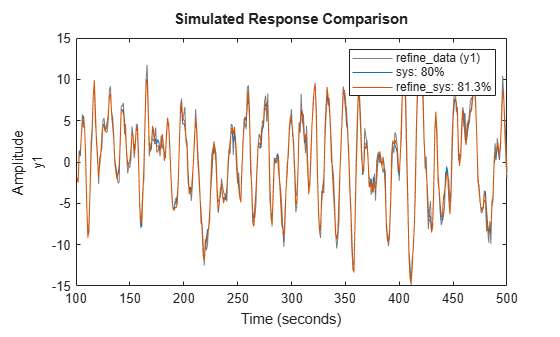Main Content

# Refine Initial ARMAX Model at Command Line

This example shows how to estimate an initial model and refine it using `pem`.

Load measured data.

`load iddata8`

Split the data into an initial estimation data set and a refinement data set.

```init_data = z8(1:100); refine_data = z8(101:end);```

`init_data` is an `iddata` object containing the first 100 samples from `z8` and `refine_data` is an `iddata` object representing the remaining data in `z8`.

Estimate an ARMAX model.

```na = 4; nb = [3 2 3]; nc = 2; nk = [0 0 0]; sys = armax(init_data,[na nb nc nk]);```

`armax` uses the default algorithm properties to estimate `sys`.

Refine the estimated model by specifying the estimation algorithm options. Specify stricter tolerance and increase the maximum iterations.

```opt = armaxOptions; opt.SearchOptions.Tolerance = 1e-5; opt.SearchOptions.MaxIterations = 50; refine_sys = pem(refine_data,sys,opt);```

Compare the fit of the initial and refined models.

`compare(refine_data,sys,refine_sys)``refine_sys` provides a closer fit to the data than `sys`.

You can similarly use `polyest` or `armax` to refine the estimated model.

## SupportGet trial now